How Cheenta works to ensure student success?
Explore the Back-Story

# AMC 8 2020 Problem 18 | Area ProblemTry this beautiful Problem based on area from AMC 8 2020.

## Probability Problem - AMC 8 2020 Problem 18

Rectangle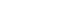is inscribed in a semicircle with diameter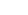, as shown in the figure. Let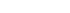, and let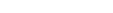. What is the area of?

• 240
• 248
• 256
• 264
• 272

Area

Semi circle

Symmetry

## Suggested Book | Source | Answer

AMC 8 2020 Problem 13

240

## Try with Hints

Try to find the diameter of the semicircle. So the diameter will be,

The diameter of the semicircle is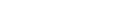, so. By symmetry,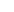is the midpoint of AD,So,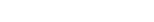.

Now, apply Pythagorean Theorem to find CD,

SO the area of ABCD will be=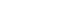AMC-AIME Program at Cheenta

## Subscribe to Cheenta at Youtube

Try this beautiful Problem based on area from AMC 8 2020.

## Probability Problem - AMC 8 2020 Problem 18

Rectangleis inscribed in a semicircle with diameter, as shown in the figure. Let, and let. What is the area of?

• 240
• 248
• 256
• 264
• 272

Area

Semi circle

Symmetry

## Suggested Book | Source | Answer

AMC 8 2020 Problem 13

240

## Try with Hints

Try to find the diameter of the semicircle. So the diameter will be,

The diameter of the semicircle is, so. By symmetry,is the midpoint of AD,So,.

Now, apply Pythagorean Theorem to find CD,

SO the area of ABCD will be=AMC-AIME Program at Cheenta

## Subscribe to Cheenta at Youtube

This site uses Akismet to reduce spam. Learn how your comment data is processed.

### Knowledge Partner